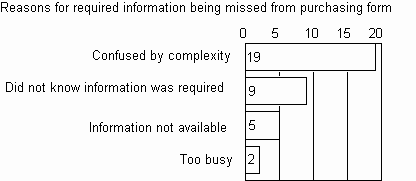The Psychology of Quality and More

Pareto Chart: Practical variations

The Quality Toolbook > Pareto Chart > Practical variations

Practical variations

• A common addition is a cumulative line, which adds the height of each bar in turn. A right-hand scale can be used to show the cumulative line as a percentage of the total of all measurements, as below.Fig. 1. Cumulative Pareto Curve

• Take the 80/20 rule literally, and draw a line across from the 80% mark to the cumulative line and then down into the bars, as in the diagram above. Select all bars which are completely or partially to the left of this line. This gives a simple rule-based selection procedure.
• When a cumulative line is used, the bars may be omitted. This line is sometimes called a Pareto curve.
• Turn chart on its side, to allow horizontal text for bar labels. This is particularly useful when the labels are long, as in the illustration below.
• Draw scale lines across the chart to help estimate the values on each bar, as in the figure below.
• Add numbers to bars to show exact values. Either put them inside the bar or on top of the bar, as below.Fig. 2. Horizontal Pareto, with numbering

• In ABC Analysis, the cumulative figures are divided not only at 20%, but also at 50%. This gives three areas (A, B and C) which are expected to contain 80%, 15% and 5% of the problems, respectively.
• Compare the current Pareto Chart figures with a previous set of measurements using either a Pareto Pyramid or a Paired Pareto Chart, as below.Fig. 3. Pareto Pyramid and Paired Pareto Chart

You can buy books here

And the big
paperback book